# Activation Functions in Neural Networks: With 15 examples

Nikolaj Buhl
July 25, 2023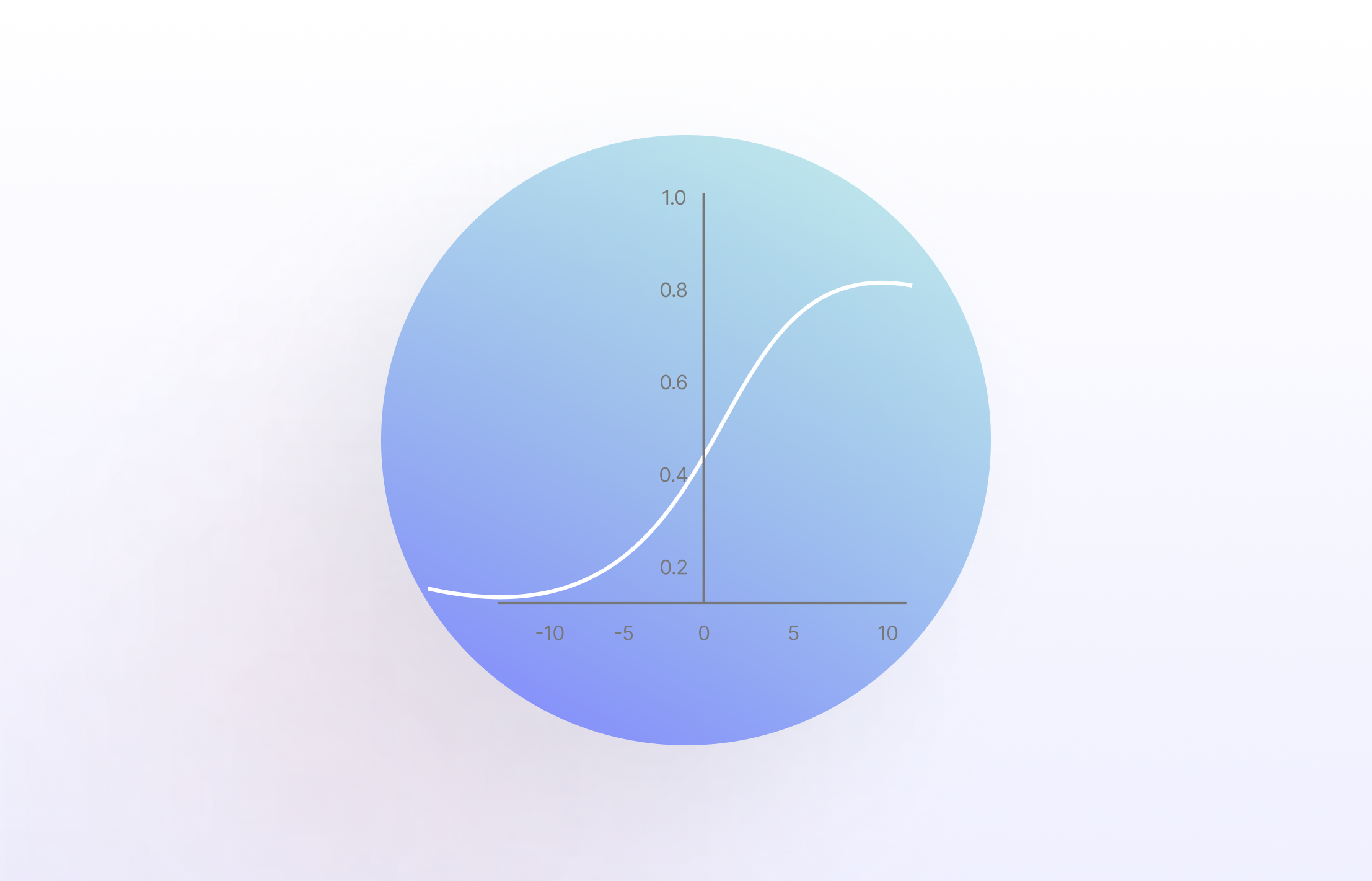Activation functions play a crucial role in neural networks, performing a vital function in hidden layers to solve complex problems and to analyze and transmit data throughout deep learning algorithms. There are dozens of activation functions, including binary, linear, and numerous non-linear variants.

The activation function defines the output of a node based on a set of specific inputs in machine learning, deep neural networks, and artificial neural networks.

Activation functions in artificial neural networks are comparable to cells and neurons in the human brain. Data science is working hard to give computers the ability to think as close as possible to humans so that one day artificial intelligence might be able to think for itself creatively, getting closer to passing the Turing test

Accelerate your machine learning project 10x with Encord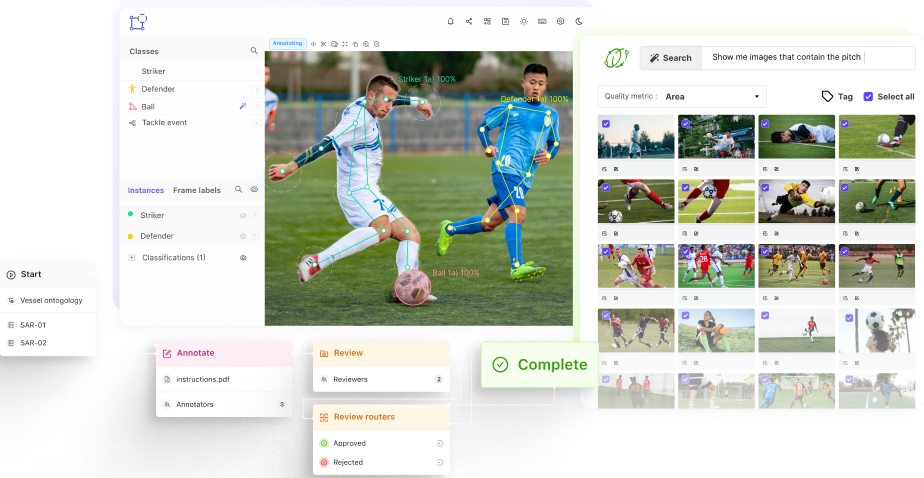When you are driving, there are numerous tasks to concentrate on. As you approach a traffic light, you may recall additional items to include in your shopping list or your children’s permission slip that requires signing. Does that mean you stop concentrating and crash the car?

No, it does not. The human brain is a powerful computational engine and can hold multiple thoughts, inputs, and outputs at the same time without compromising decision-making, reflexes, and reasoning.

Within neural networks and any algorithmic models, the aim is to develop a system as capable ⏤ if not more, in many cases ⏤ as the human brain. Activation functions are designed to open up a neural network's problem-solving abilities.

Activation functions ensure algorithmic networks (e.g., neural, deep learning, artificial intelligence, machine learning, convolutional neural networks, etc.) focus on priority problems by splitting or segregating the inputs to ensure processing power is being used most effectively.

💡 The aim of neural network activation functions: In order to get access to a much richer hypothesis space that would benefit from deep representations, you need a non-linearity, or activation function.” ⏤ Deep Learning with Python, 2017, page 72

## What Are Neural Network Activation Functions?

Neural networks are machine learning models that are inspired by and, in many ways, modeled on the structure and functionalities of the human brain. They are composed of layers of interconnected nodes that process and transmit information, making them deep networks. Nodes are an integral part of the overall network, and we can think of them as comparable to brain cells.

Activation functions are a critical component of neural networks that introduce non-linearity into the model, allowing networks to learn complex patterns and relationships in the data. These functions play an important role in the hyperparameters of AI-based models.

There are numerous different activation functions to choose from. For data scientists and machine learning engineers, the challenge can be knowing which function or series of functions to use to train a neural network.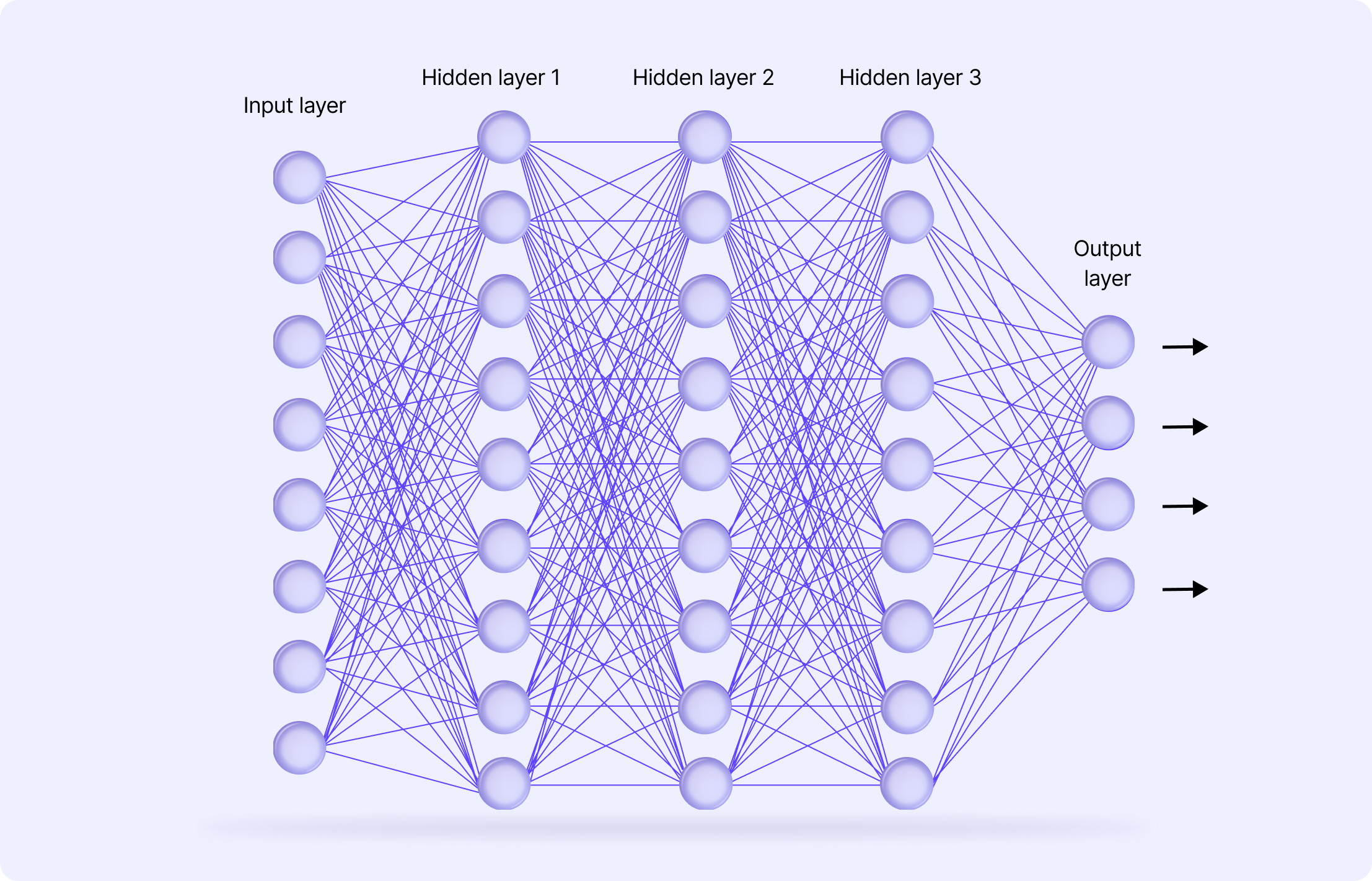In this article, we explore 15 examples of activation functions and how they can be used to train neural networks.

## Why Neural Networks Need Activation Functions?

Activation functions are necessary for neural networks because, without them, the output of the model would simply be a linear function of the input. In other words, it wouldn’t be able to handle large volumes of complex data. Activation functions are an additional step in each forward propagation layer but a valuable one.

💡 Without nonlinearity, a neural network would only function as a simple linear regression model.

Even if there were multiple layers, neurons, or nodes in the network, problems wouldn’t be analyzed between one layer and the next layer without activation functions. Data scientists often test different activation functions when designing a model, and aim for maximum optimization of the one being deployed.

Deep learning networks wouldn’t learn more complicated patterns because they all function in a linear format. This would limit the model's ability to learn complex patterns and relationships in the datasets it’s being trained on.

By introducing nonlinearity through activation functions, neural networks are able to model more complex functions within every node, which enables the neural network to learn more effectively. Before we dive into different activation functions, it helps to refresh our knowledge of the architecture of neural networks to understand better where and how activation functions play a role in model development.

## Neural Networks Architecture: Overview

To understand the role of activation functions in neural networks, it's important first to understand the basic elements of the network's architecture.

The architecture of neural networks is made of three core layers:

1. Input layer
2. Hidden layers
3. Output layer

### Input Layer

The input layer is where the raw data/datasets are received. In the case of computer vision neural network projects, this could be image or video-based datasets.

There isn’t anything complex about this layer, and there isn’t any computation at this stage. It simply serves as a gateway for those inputting the data to train a model, and then everything gets passed onto the hidden layer(s).

### Hidden Layer(s)

Complex and advanced neural networks usually have one or more hidden layers. This is where the data is processed by numerous nonlinear neurons and activation functions that each perform their own role.

In every neural network, different nodes and neurons are characterized (and perform tasks) based on their specific activation function, weight, and bias. Results from the computational energy and tasks implemented in these hidden layers are then passed onto the output layer. It’s also in these layers where optimizations can be put into practice to improve model performance and outputs.

💡 In most cases, the activation function used is applied across every hidden layer. However, the activation function found in the output layer is usually different from that found in the hidden layers. Which activation function is chosen depends on the goal or prediction type or outputs project managers and owners want a neural network to produce.

### Output Layer

The output layer produces the final series of calculations, predictions, or classifications using the input data and the outputs/results processed through the hidden layers.

It’s also worth pointing out and taking a moment to shine a light on two other terms that are integral to the architecture of neural networks: feedforward and backpropagation.

### Feedforward vs. Backpropagation

In feedforward networks, also known as forward propagation, the flow of data moves forward, as the name suggests in feedforward neural networks. In these networks, the activation functions operate as a mathematical gate that sits between the input feeding data into the current neuron or node in the network.

On the other hand, backpropagation adjusts a network's weights and biases using gradients to minimize the cost. This determines the level of adjustments required to the weights, biases, and activation functions. These adjustments are then propagated backward through the network to minimize the gap between the actual output vector and target outputs.

Now let’s take a closer look at 15 of the most popular and useful activation functions in neural networks.

## 15 Types of Neural Networks Activation Functions

Activation functions can generally be classified into three main categories: binary step, linear, and non-linear, with numerous subcategories, derivatives, variations, and other calculations now being used in neural networks.

Binary step is the simplest type of activation function, where the output is binary based on whether the input is above or below a certain threshold. Linear functions are also relatively simple, where the output is proportional to the input. Non-linear functions are more complex and introduce non-linearity into the model, such as Sigmoid and Tanh.

In every case, the activation function is picked based on the specific problem and challenge that needs solving. It isn’t always obvious which one data scientists and machine learning engineers need to use, so sometimes it’s a case of trial and error.  But that’s always the starting point for choosing the right activation function for a neural network or any other kind of complicated algorithmic-based model that requires activation functions.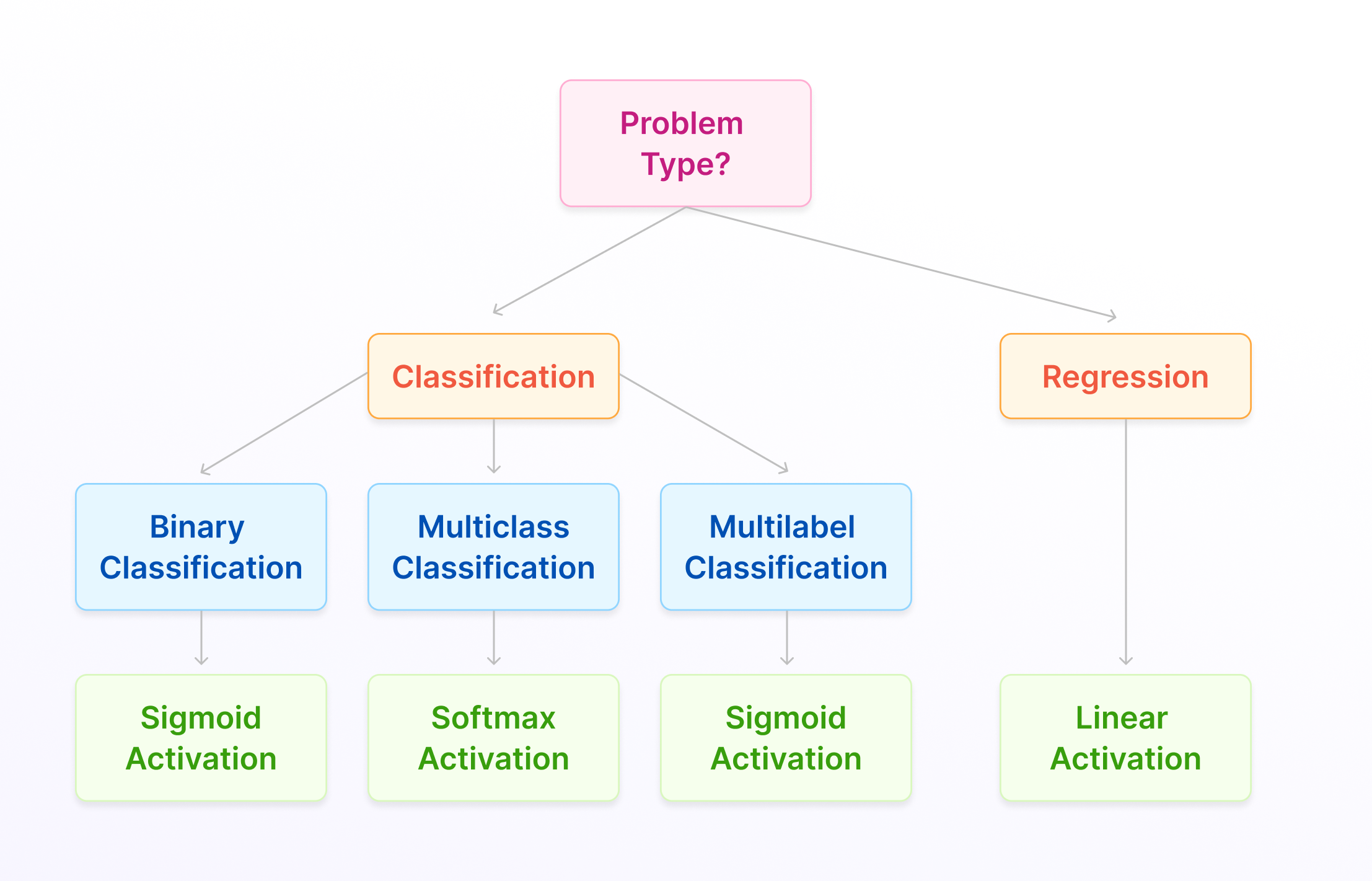Here are 15 activation functions in more detail, with the majority being non-linear.

## Linear Activation Functions

Let’s start with the linear functions before going onto the non-linear functions.

### Linear Activation Function (Identity)

In deep learning, data scientists use linear activation functions, also known as identity functions, when they want the output to be the same as the input signal. Identity is differentiable, and like a train passing through a station without stopping, this activation function doesn’t change the signal in any way, so it’s not used within internal layers of a DL network.

Although, in most cases, this might not sound very useful, it is when you want the outputs of your neural network to be continuous rather than modified or discrete. There is no convergence of data, and nothing decreases either. If you use this activation function for every layer, then it would collapse the layers in a neural network into one. So, not very useful unless that’s exactly what you need or there are different activation functions in the subsequent hidden layers.

Here is the mathematical representation: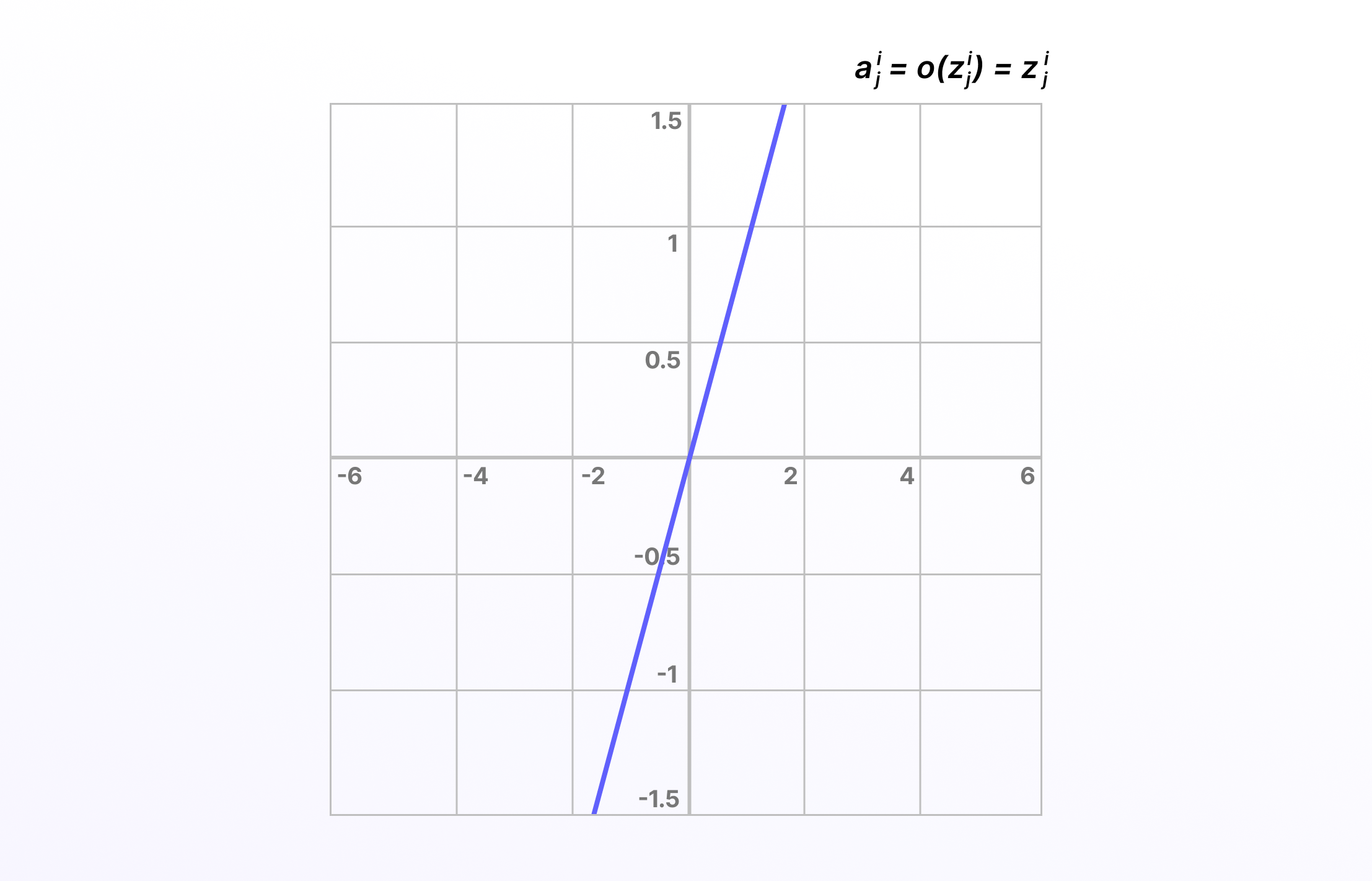### Piecewise Linear (PL)

Piecewise linear is an iteration on the above, except involving an affine function, so it is also known as piecewise affine. It’s defined using a bound or unbound sequence of numbers, either compact, finite, or locally finite, and is not differentiable due to threshold points, so it only propagates signals in the slope region.

Piecewise linear is calculated using a range of numbers required for the particular equation, anything less than the range is 0, and anything greater is 1. Between 0 and 1, the signals going from one layer to the next are linearly-interpolated.

Here is the mathematical representation: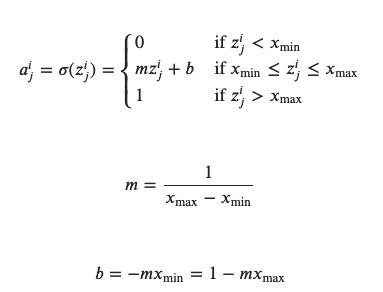Linear activation functions don’t allow neural networks or deep learning networks to develop complex mapping and algorithmic interpretation between inputs and outputs.

## Non-Linear Activation Functions

Non-linear activation functions solve the limitations and drawbacks of simpler activation functions, such as the vanishing gradient problem. Non-linear functions, such as Sigmoid, Tanh, Rectified Linear Unit (ReLU), and numerous others.

There are several advantages to using non-linear activation functions, as they can facilitate backpropagation and stacking. Non-linear combinations and functions used throughout a network mean that data scientists and machine learning teams creating and training a model can adjust weights and biases, and outputs are represented as a functional computation.

In other words, everything going into, through, and out of a neural network can be measured more effectively when non-linear activation functions are used, and therefore, the equations are adjusted until the right outputs are achieved.

💡Explore advances in neural network motion estimation that relies on activation functions: Tracking Everything Everywhere All at Once | Explained

### Binary Step Function

The binary step function is a door that only opens when a specific threshold value has been met. When an input is above that threshold, the neuron is activated, and when not, it’s deactivated.

Once a neuron is activated then, the output from the previous layer is passed onto the next stage of the neural network’s hidden layers.

Binary step is purely threshold-based, and of course, it has limitations, such as it not being differentiable and it can’t backpropogate signals. It can’t provide multi-value outputs or multi-class classification problems when there are multiple outputs.

However, for fairly simple neural networks, the binary step is a useful and easy activation function to incorporate.

Here is the mathematical representation: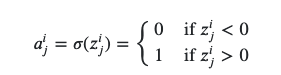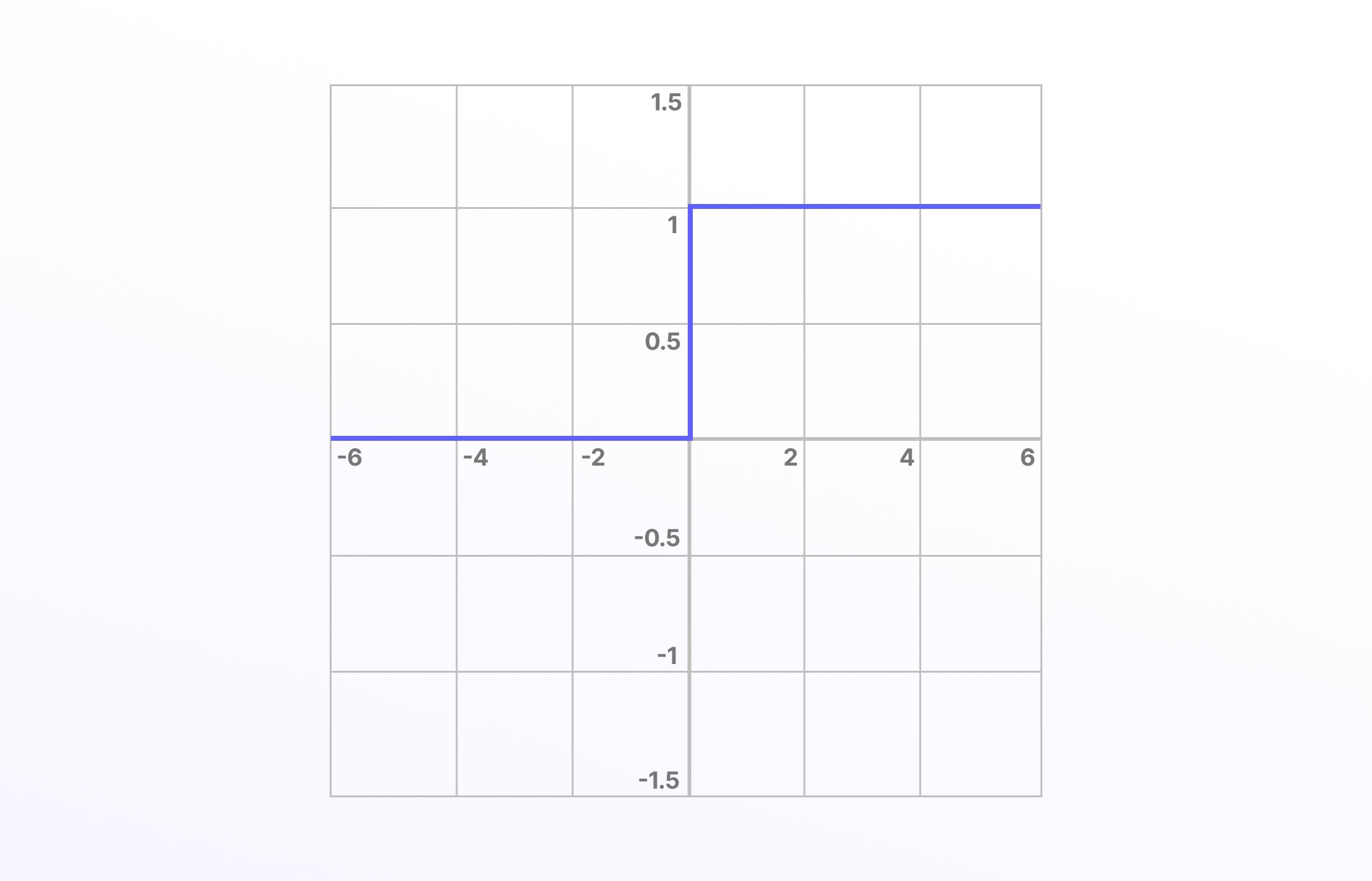### Sigmoid, Logistic Activation Functions

‌The Sigmoid activation function, also known as the logistic activation function, takes inputs and turns them into outputs ranging between 0 and 1. For this reason, sigmoid is referred to as the “squashing function” and is differentiable. Larger, more positive inputs should produce output values close to 1.0, with smaller, more negative inputs producing outputs closer to 0.0.

It’s especially useful for classification or probability prediction tasks so that it can be implemented into the training of computer vision and deep learning networks. However, vanishing gradients can make these problematic when used in hidden layers, and this can cause issues when training a model.

Here is the mathematical representation: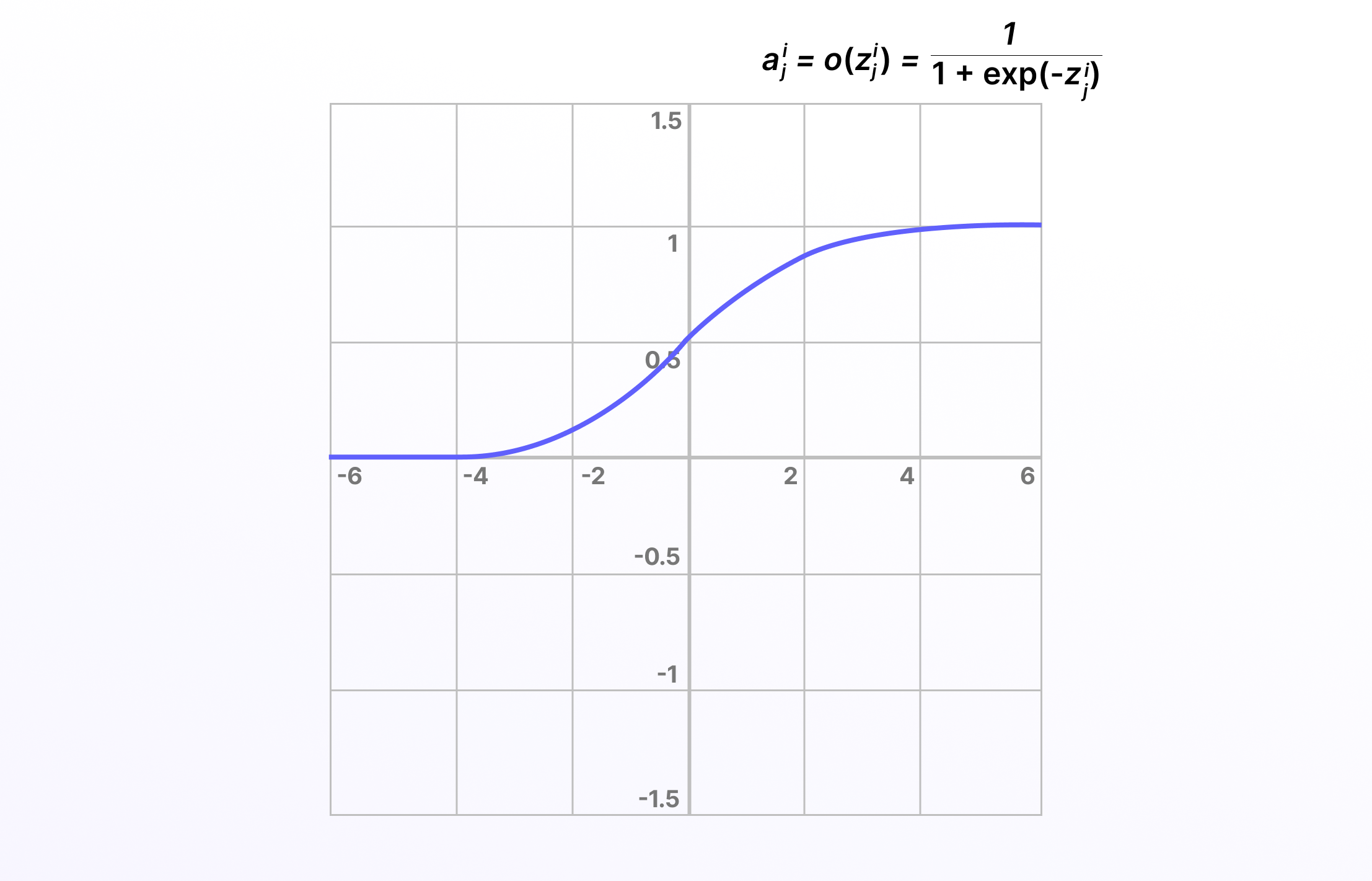### Tanh Function (Hyperbolic Tangent)

Tanh (or TanH), also known as the hyperbolic tangent activation function, is similar to sigmoid/logistic, even down to the S shape curve, and it is differentiable. Except, in this case, the output range is -1 to 1 (instead of 0 to 1). It is a steeper gradient and also encounters the same vanishing gradient challenge as sigmoid/logistic.

Because the outputs of tanh are zero-centric, the values can be more easily mapped on a scale between strongly negative, neutral, or positive.

Here is the mathematical representation: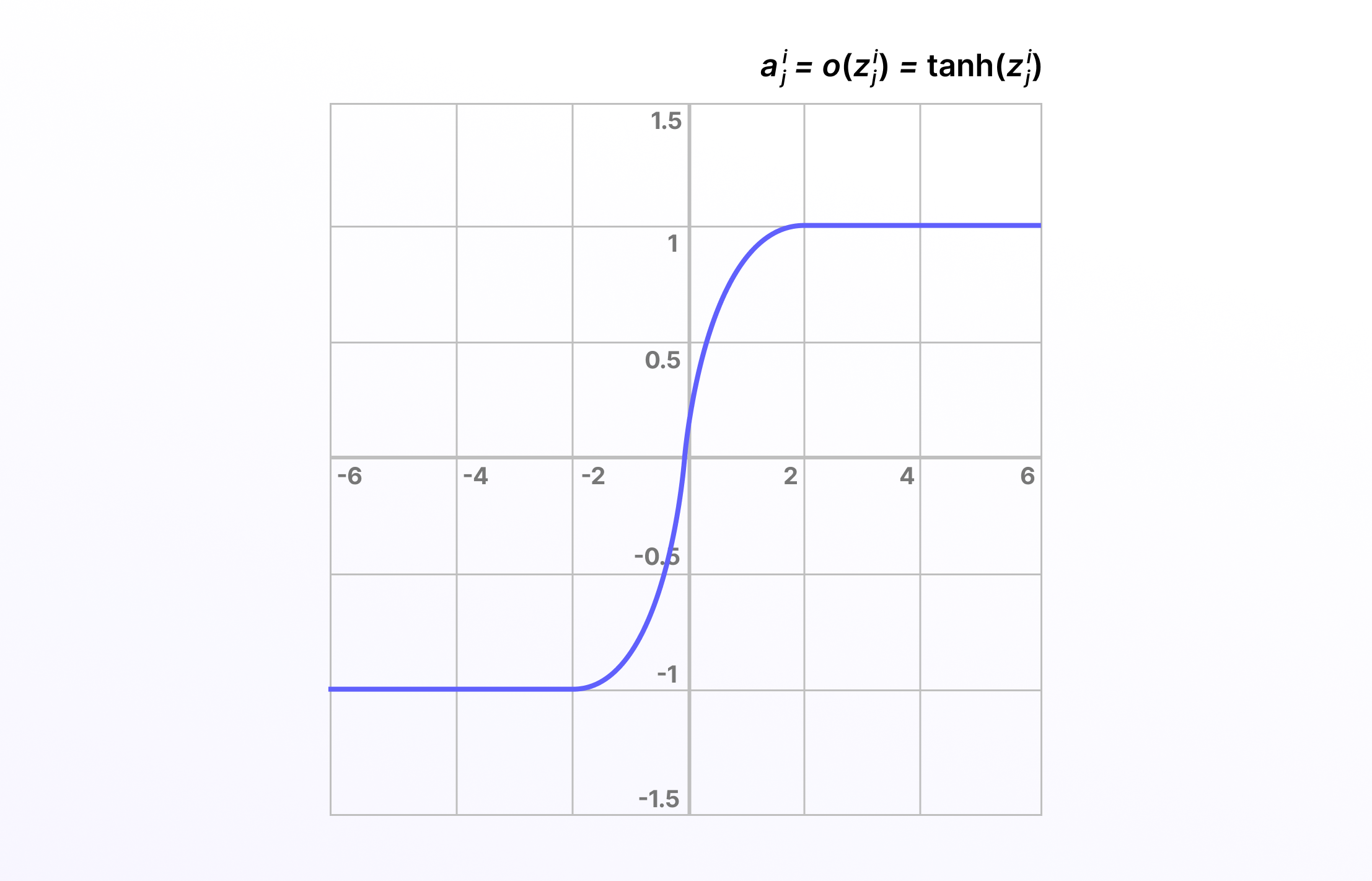### Rectified Linear Unit (ReLU)

Compared to linear functions, the rectified linear unit (ReLU) is more computationally efficient For many years, researchers and data scientists mainly used Sigmoid or Tanh, and then when ReLU came along, training performance increased significantly. ReLU isn’t differentiable, but this isn’t a problem because derivatives can be generated for ReLU.

ReLU doesn’t activate every neuron in sequence at the same time, making it more efficient than the tanh or sigmoid/logistic activation functions. Unfortunately, the downside of this is that some weights and biases for neurons in the network might not get updated or activated.

This is known as the “dying ReLU” problem, and it can be solved in a number of ways, such as using variations on this formula, including the exponential ReLU or parametric ReLU function.

Here is the mathematical representation: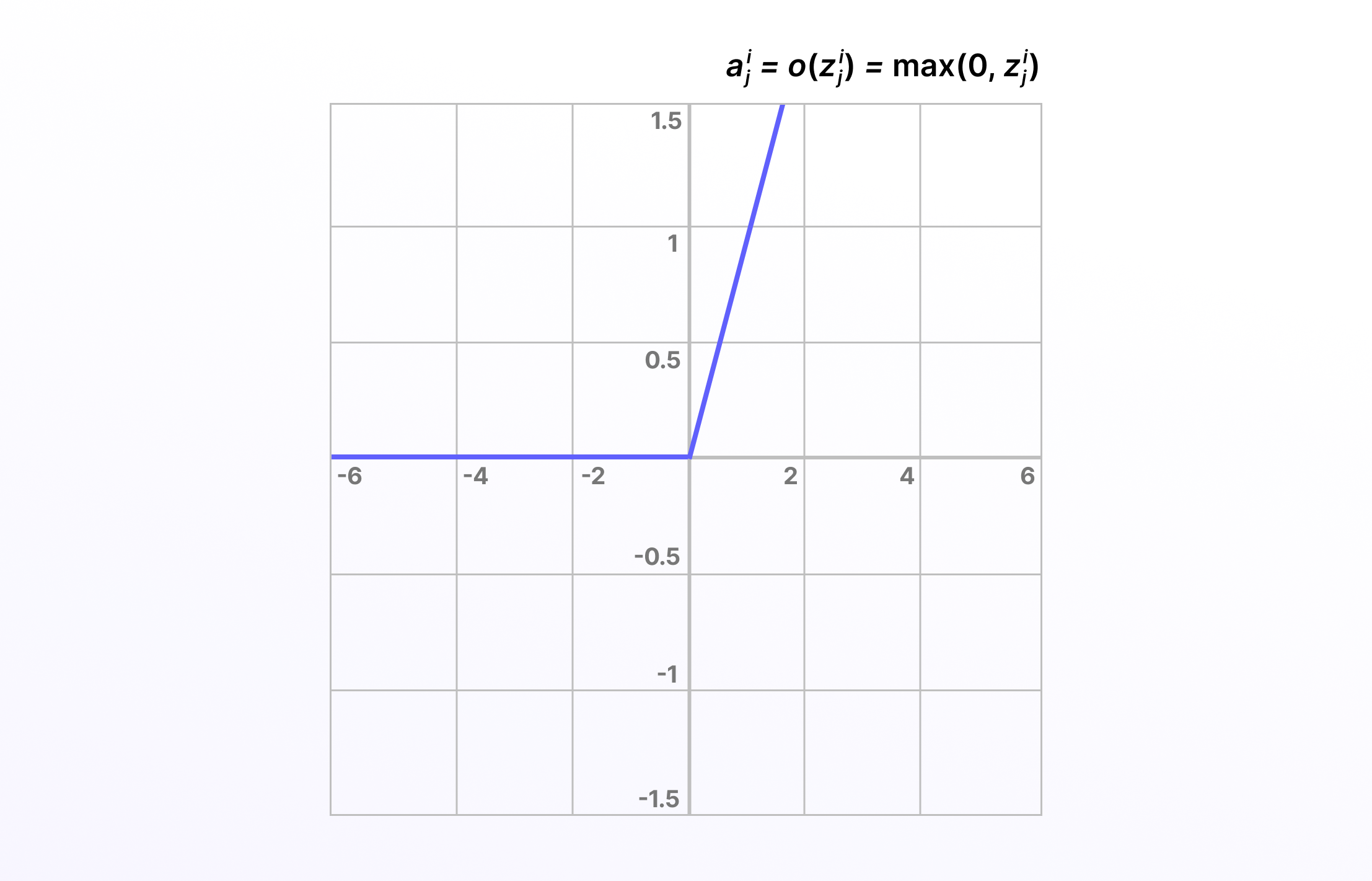### Leaky ReLU Function

One solution to the “dying ReLU” problem is a variation on this known as the Leaky ReLU activation function. With the Leaky ReLU, instead of being 0 when 𝑧<0, a leaky ReLU allows a small, non-zero, constant gradient 𝛼 (Normally, 𝛼=0.01).

Here is the mathematical representation: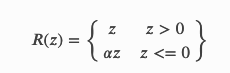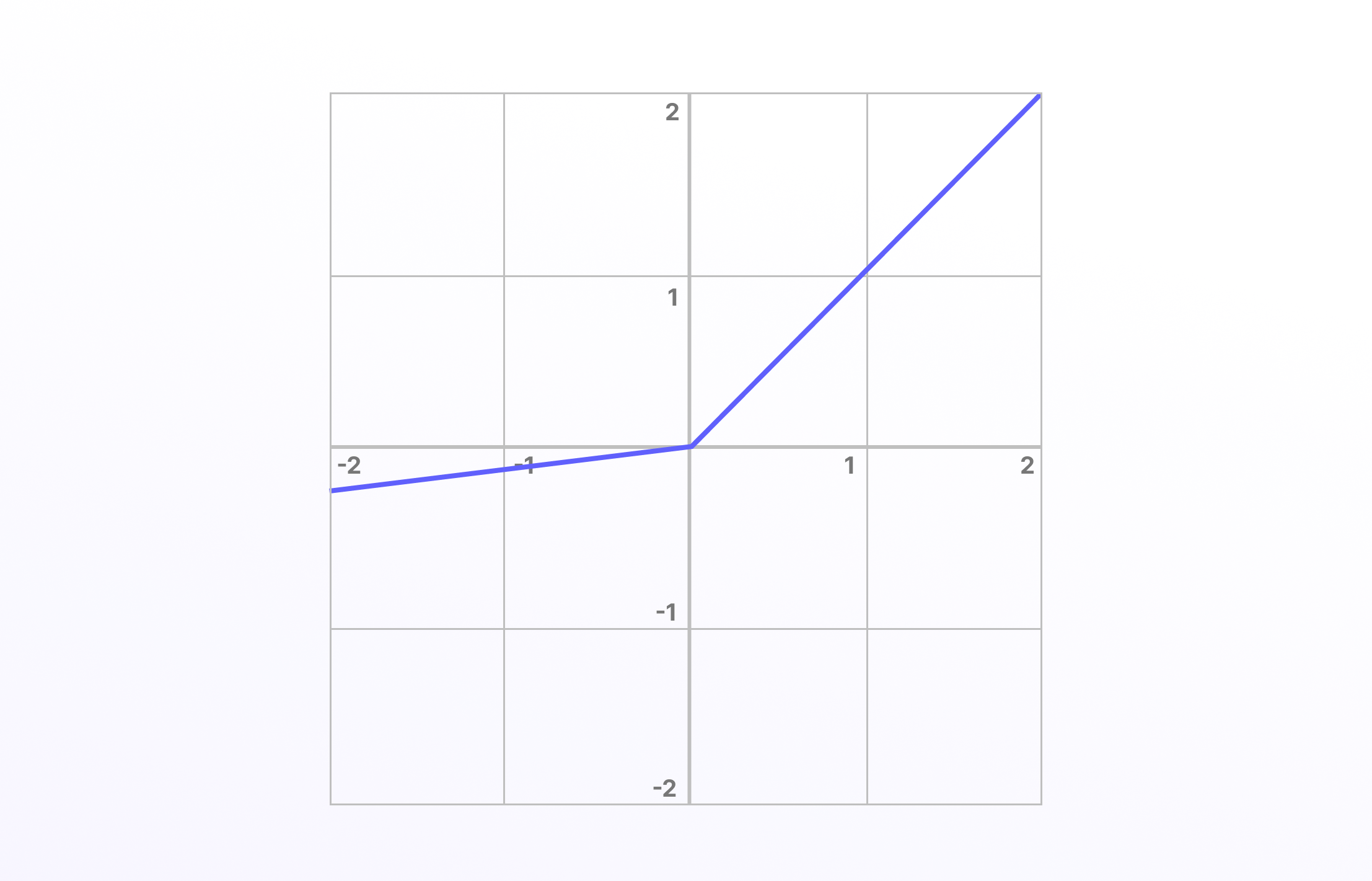Leaky ReLU has been shown to perform better than the traditional ReLU activation function. However, because it possesses linearity it can’t be used for more complex classification tasks and lags behind more advanced activation functions such as Sigmoid and Tanh.

### Parametric ReLU Function

Parametric ReLU is another iteration of ReLU (an advance on the above, Leaky ReLU) except with a parameterized slope α, and is also not differentiable.

Again, this activation function generally outperforms ReLU especially when used for image classification tasks in deep learning. Parametric ReLU reduces the number of parameters required to achieve higher levels of performance and is a feature of numerous deep learning architectures and models such as ResNet, DenseNet, and Alexnet.

Here is the mathematical representation: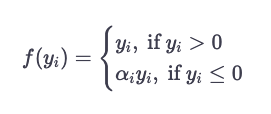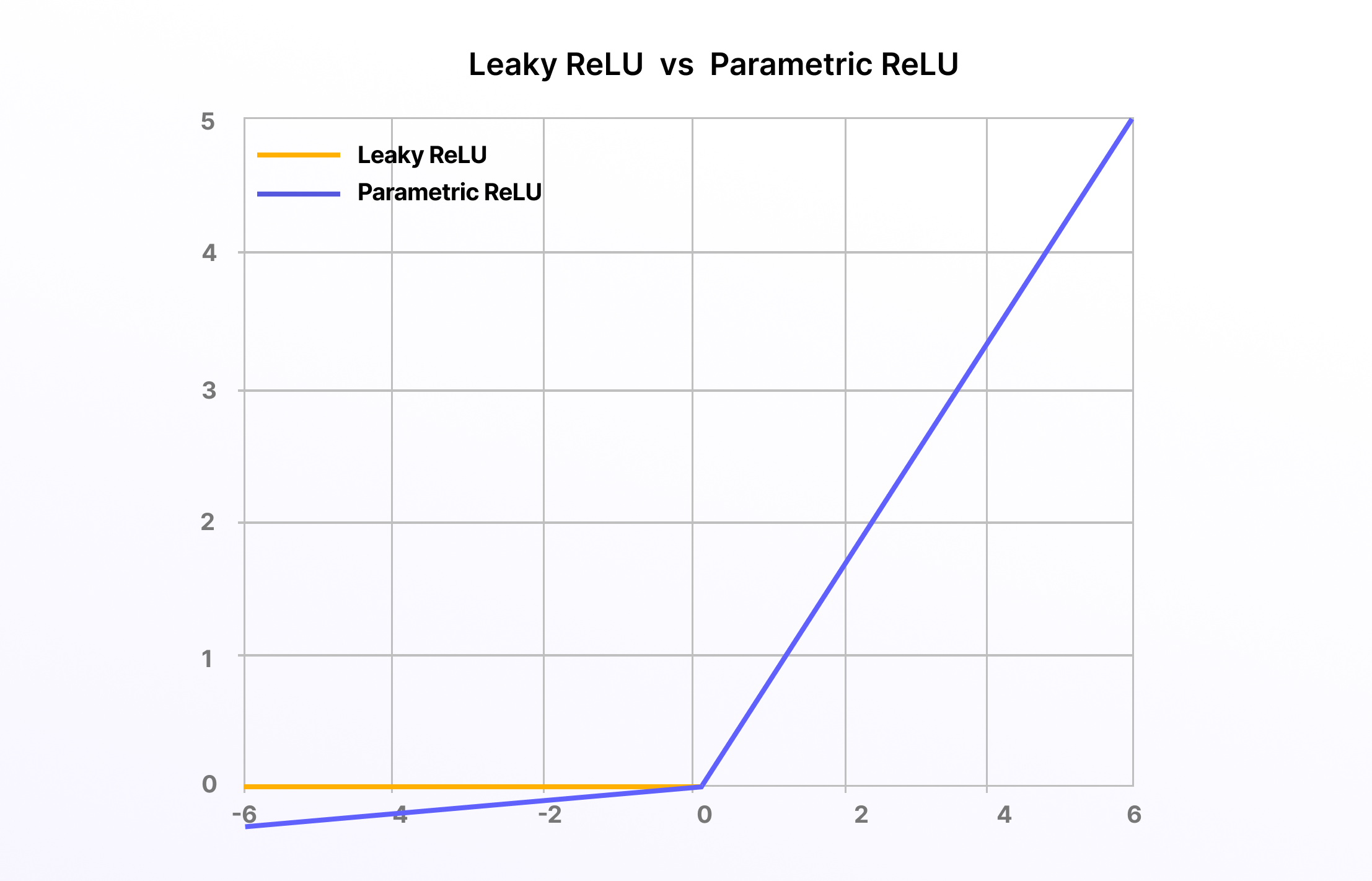💡 Learn more about the Parametric ReLU Function in the Microsoft Research paper by Kaiming He et al. (2015): “Delving Deep into Rectifiers: Surpassing Human-Level Performance on ImageNet Classification.”

### Exponential Linear Units (ELUs) Function

The exponential linear units (ELUs) function is another iteration on the original ReLU, another way to overcome the “dying ReLU” problem, and it’s also not differentiable. ELUs use a log curve for negative values instead of a straight line, with it becoming smooth slowly until it reaches -α.

Here is the mathematical representation: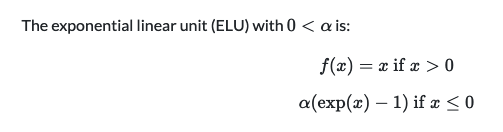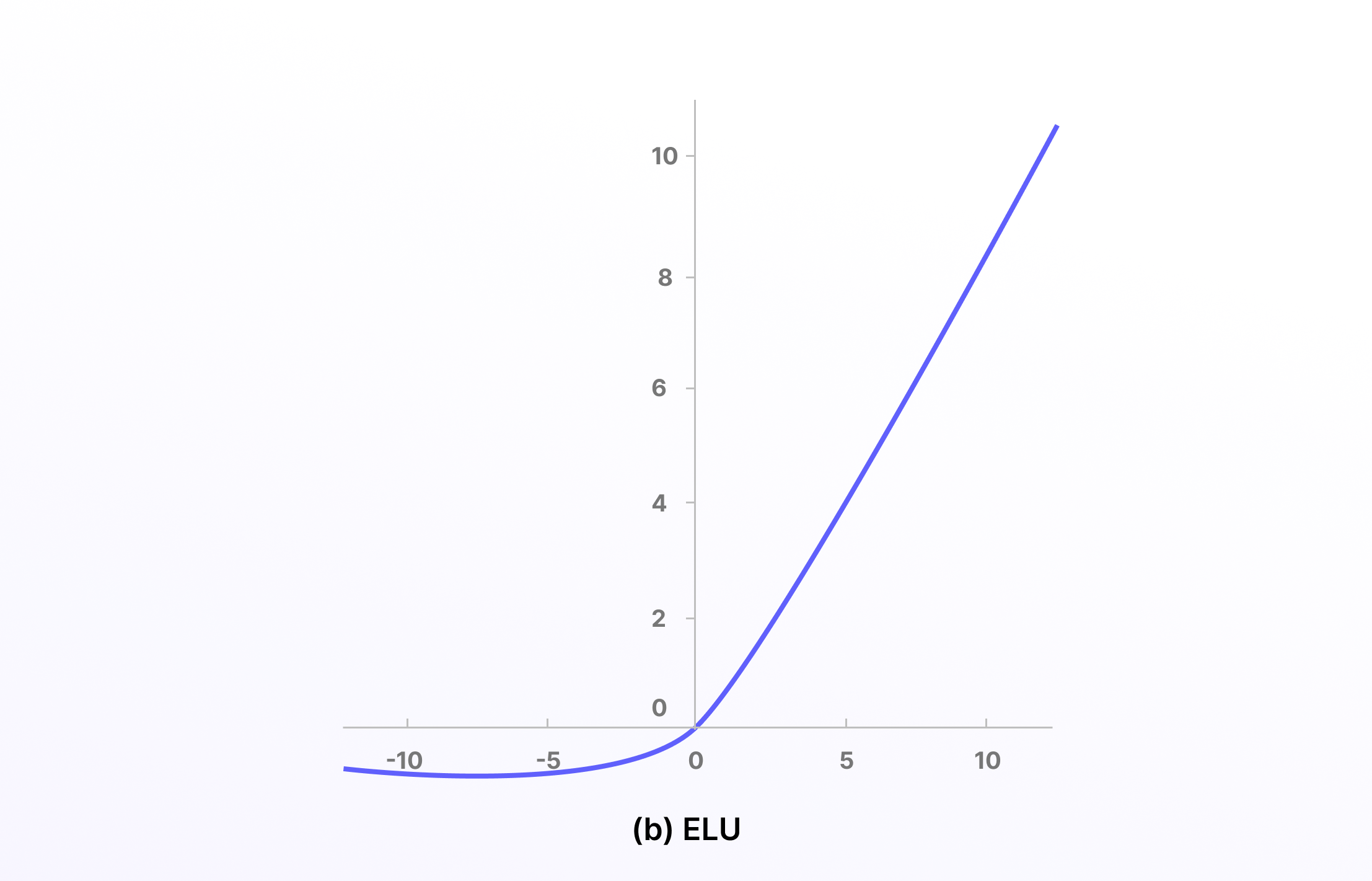### Scaled Exponential Linear Units (SELUs)

Scaled exponential linear units (SELUs) first appeared in this 2017 paper. Similar to ELUs, the scaled version of this is also attempting to overcome the same challenges of ReLUs.

SELUs control the gradient more effectively and scale the normalization concept, and that is scales with a lambda parameter. SELUs remove the problem of vanishing gradients, can’t die (unlike ReLUs), and learn faster and better than other more limited activation functions.

Here is the mathematical representation: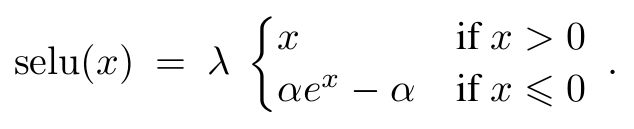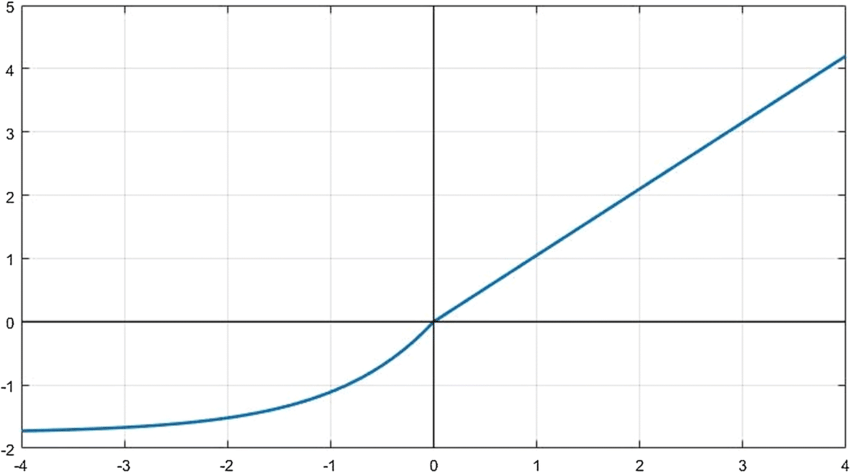💡Take a closer look at Meta’s latest open-source AI offering: ImageBind MultiJoint Embedding Model from Meta Explained

### Gaussian Error Linear Units (GELUs)

Now we get into an activation function that’s compatible with top, mass-scale natural language processing (NLPs) and large language models (LLMs) like ChatGPT-3, BERT, ALBERT, and ROBERTa.

Gaussian error linear units (GELUs) are part of the Gaussian function mathematical family. GELUs combines properties and inspiration from ReLUs, dropout, and zoneout and is considered a smoother version of ReLU. You can read the paper here. Here’s what it looks like and the mathematical representation: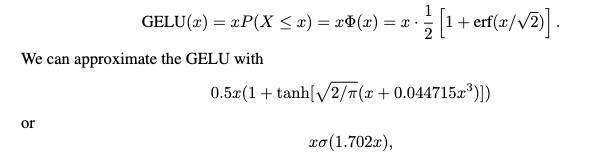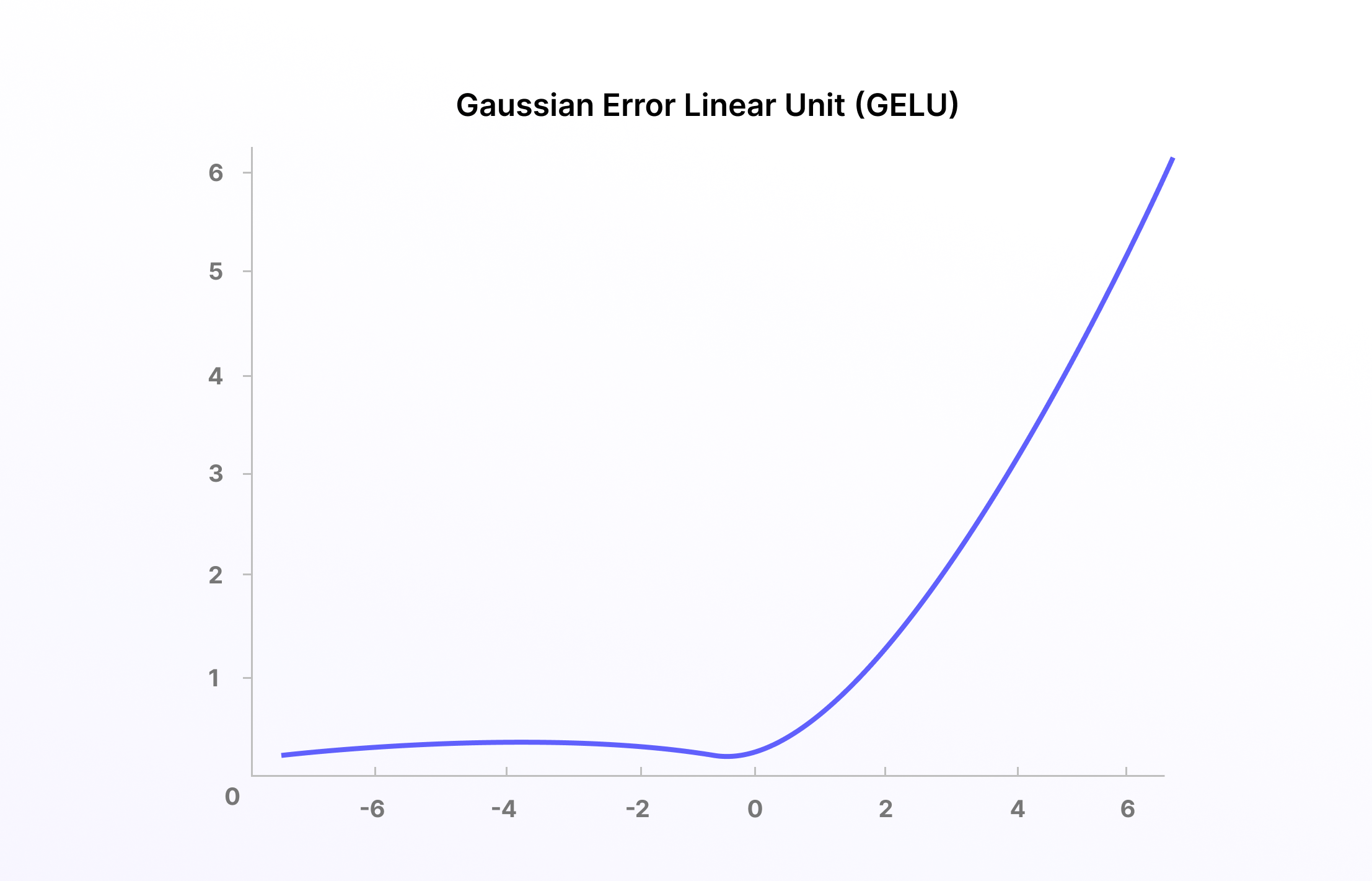### Soft Sign

Soft sign is equally useful in statistics and other related fields. It’s a continuous and differentiable activation function with a range from -1 to 1, so it can be used to model bipolar data while being computationally efficient.

Soft sign is often applied to find the maximum likelihood estimation (MLE) when data scientists are searching for other suitable activation functions that fit the training data being used.

Here is the mathematical representation: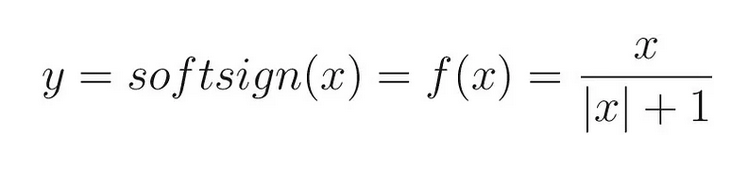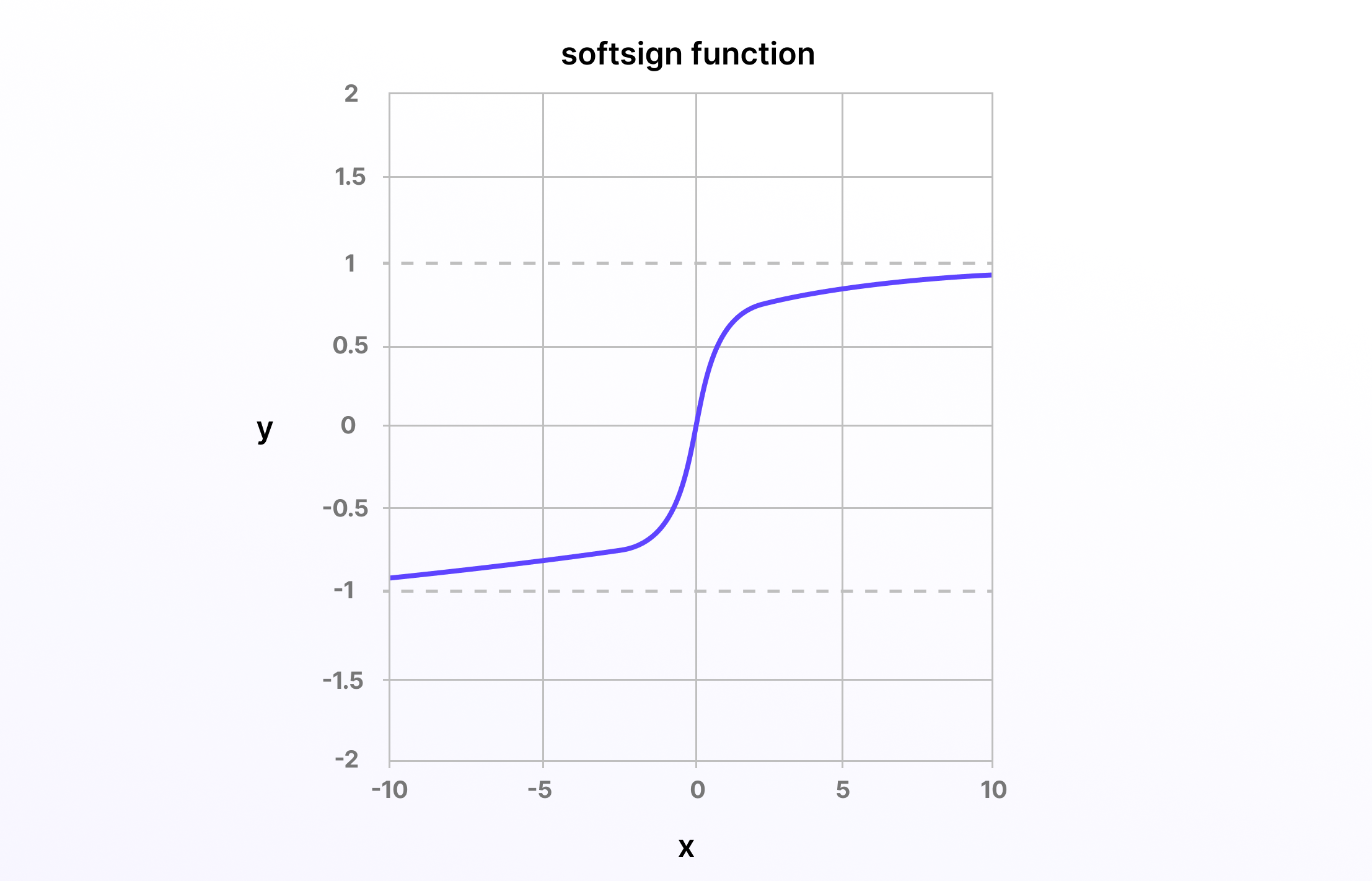### Soft Plus

Soft Plus takes Soft Sign a little further, making it an equally, if not even more, useful activation function for neural networks. Soft Plus is mathematically represented as:

f(x)=ln(1+e^x)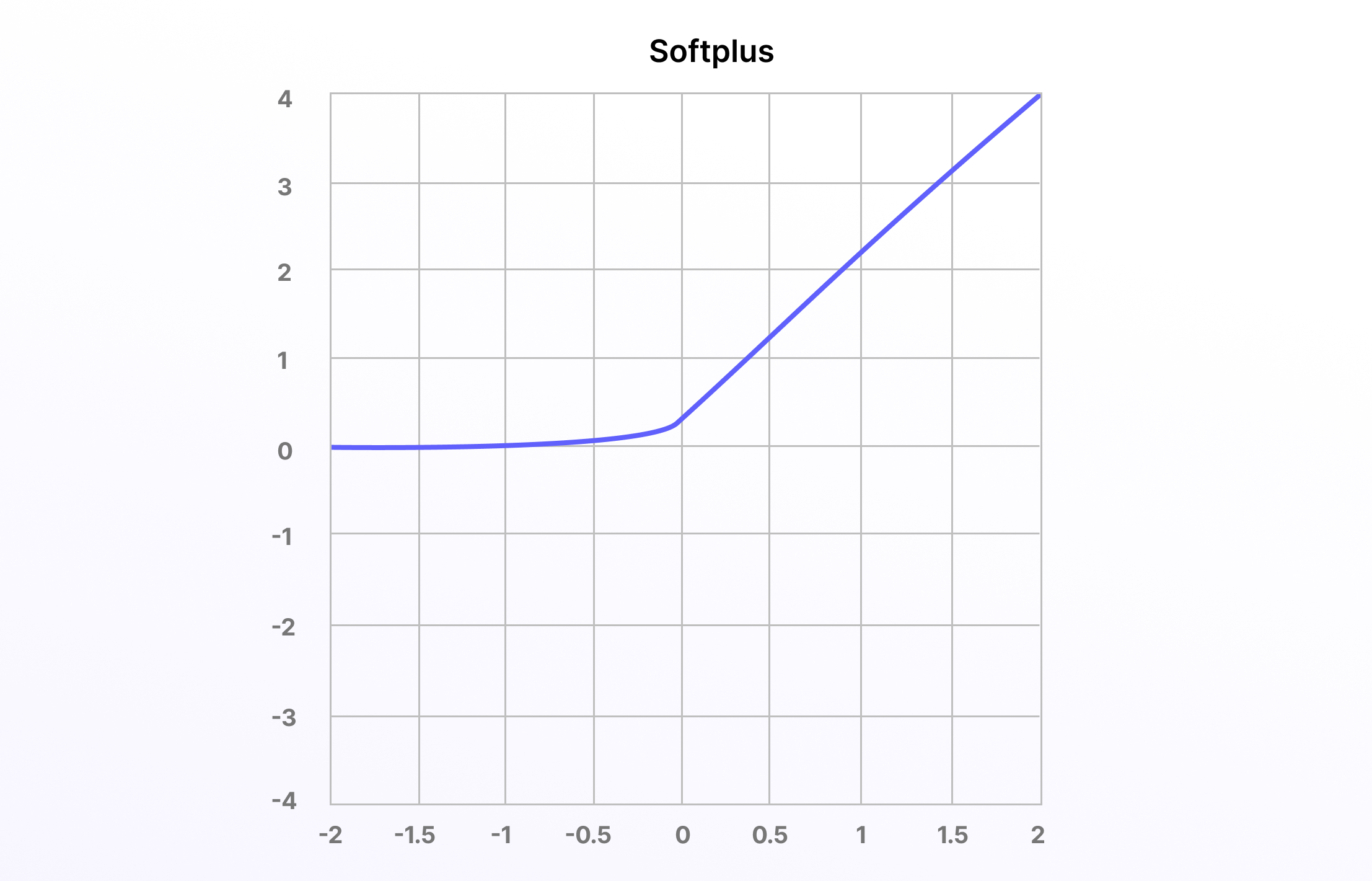Soft plus is also differentiable while being bounded and monotonic.

💡Here is another exciting AI development from Meta that leverages neural networks and activation functions: Meta AI’s I-JEPA, Image-based Joint-Embedding Predictive Architecture, Explained

### Probit

Last on this list (although there are many more; e.g., Leaky ReLU, Softmax, etc.) is probit, a quantile function that’s associated with the standard normal distribution and works as an activation function in neural networks and machine learning models.

Probit started life as a “probability unit” in statistics in 1934, first introduced by Chester Ittner Bliss.

Here is the mathematical representation: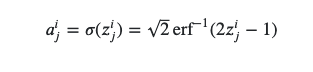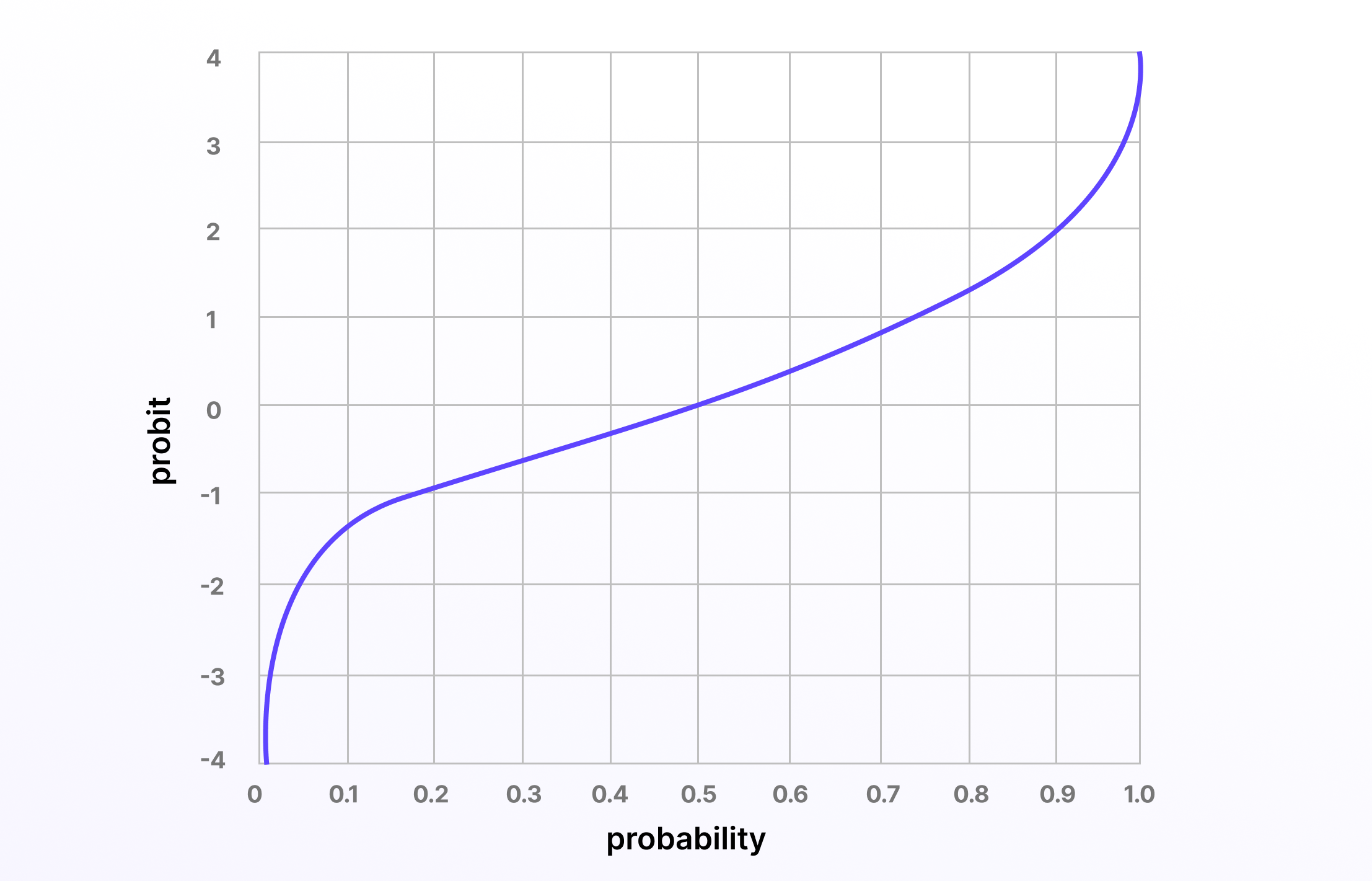### Softmax

The softmax function, also known as the softargmax function and the multi-class logistic regression, is one of the most popular and well-used differentiable layer activation functions.

Softmax turns input values that are positive, negative, zero, or greater than one into values between 0 and 1. By doing this, it turns input scores into a normalized probability distribution, making softmax a useful activation function in the final layer of deep learning and artificial neural networks.

Here is the mathematical representation: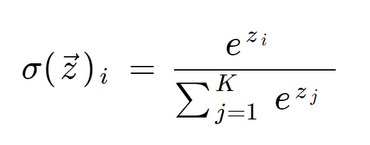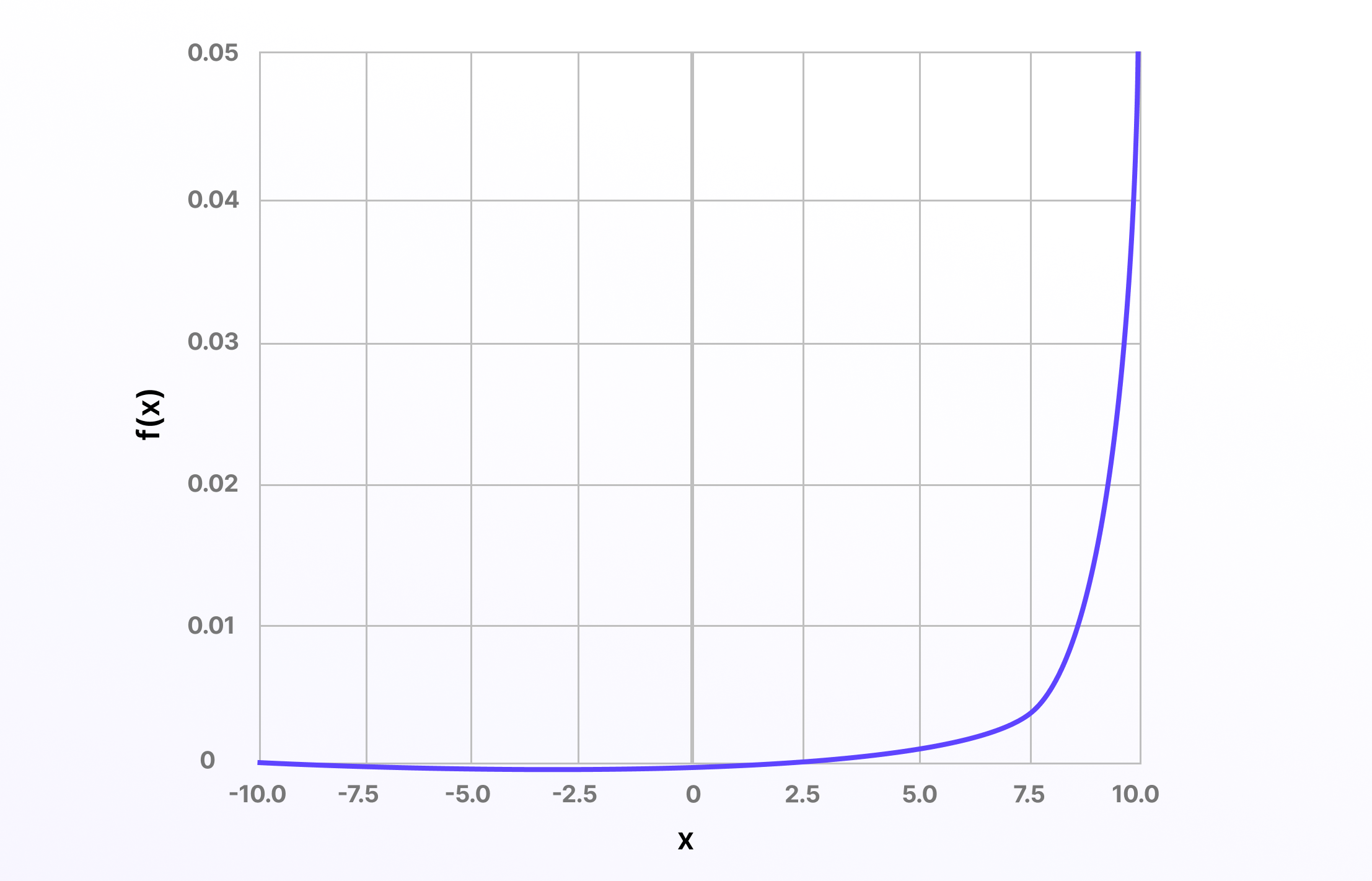## How to choose the right activation function for your ML or Computer Vision project?

Choosing the right activation function for a given machine learning or computer vision project depends on a variety of factors, including the nature of the data and the specific problem you are trying to solve.

In most cases, data science teams start with ReLU in the hidden layers and then use trial-and-error to pick a more suitable activation function if it doesn’t produce the desired outcomes.

Different activation functions perform better depending on the prediction problem, such as linear regression and softmax for multi-class classification. Your choice of activation function is also influenced by the neural network architecture.

A convolutional neural network (CNN) functions better with ReLU in the hidden layers or a variation of that (e.g., parametric, exponential, etc.), whereas a recurrent neural network (RNN) is better suited to sigmoid or tanh.

Here is a quick summary cheat sheet for choosing an activation function for your machine learning or computer vision project: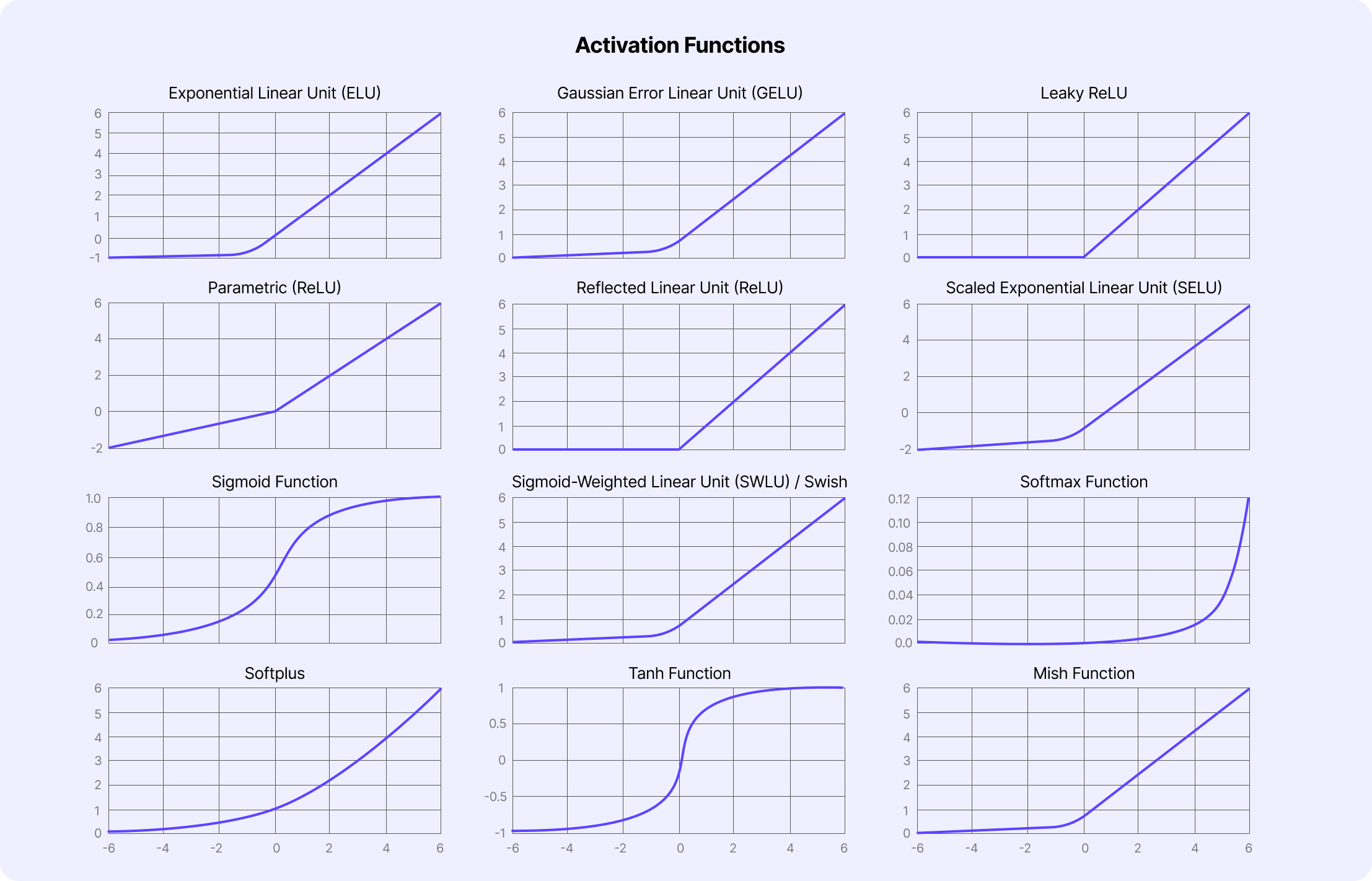Written by Nikolaj Buhl
Nikolaj is a Product Manager at Encord and a computer vision enthusiast. At Encord he oversees the development of Encord Active. Nikolaj holds a M.Sc. in Management from London Business School and Copenhagen Business School. In a previous life, he lived in China working at the Danish Embas... see more#### Discuss this blog on Slack

Join the Encord Developers community to discuss the latest in computer vision, machine learning, and data-centric AI

Join the community
###### Subscribe